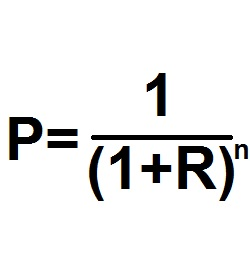Formula - Present Value | Propertylogy
Don't Miss

# Formula – Present Value

By on September 15, 2018P = [1/(1+R)n]

Where:

P = Present value

R = Periodic interest rate

n = Number of periods

The above formula helps the user discover the present value factor that is required to calculate how much is needed to be saved.

When using this factor to calculate how much money to deposit in a bank in order to attain a specific amount in future, it is important to note that the present value of 1 refers to the amount today that will be required to reach a certain amount in future.

This helps a borrower find out how much is paid or how much is required to be paid when the time elements is taken into consideration.

Mortgage analysts need to understand the dynamics of how this equation works in order to manually tabulate mortgage payments.

Otherwise, they can just use the computer.

If the deposit is \$5,000, multiplying the factor calculated from the above formula with \$5,000 will result in the amount needed to be saved.

You May Also Like...##### How to burn more calories in the office

Send this to a friend